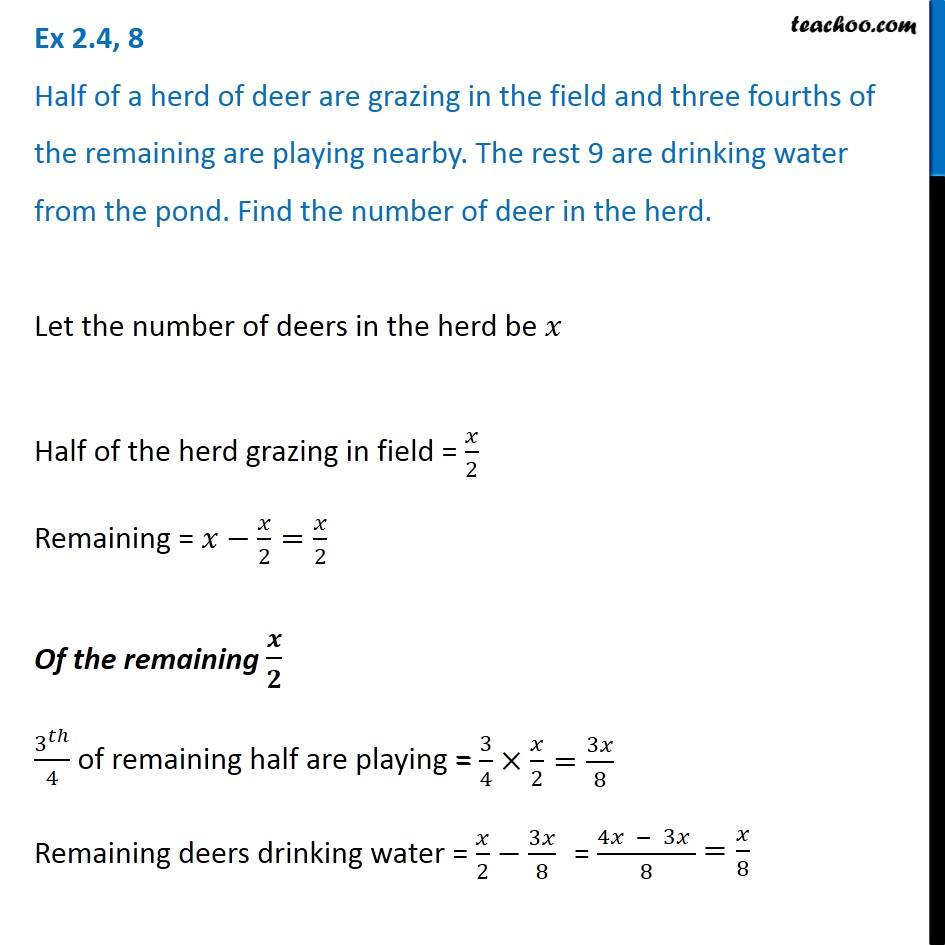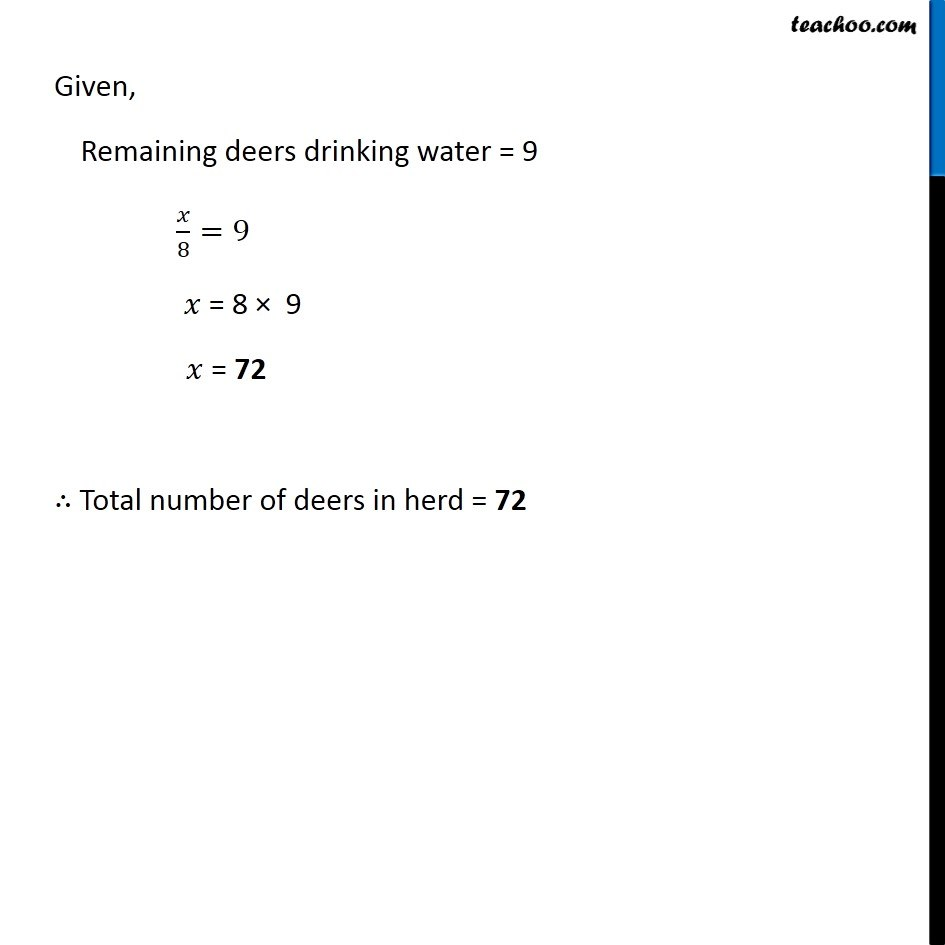Solving difficult equations

Chapter 2 Class 8 Linear Equations in One Variable
Serial order wiseLearn in your speed, with individual attention - Teachoo Maths 1-on-1 Class

### Transcript

Question 8 Half of a herd of deer are grazing in the field and three fourths of the remaining are playing nearby. The rest 9 are drinking water from the pond. Find the number of deer in the herd.Let the number of deers in the herd be 𝑥 Half of the herd grazing in field = 𝑥/2 Remaining = 𝑥−𝑥/2=𝑥/2 Of the remaining 𝒙/𝟐 3^𝑡ℎ/4 of remaining half are playing = Remaining deers drinking water = 𝑥/2−3𝑥/8 Given, Remaining deers drinking water = 9 𝑥/8=9 𝑥 = 8 × 9 𝑥 = 72 ∴ Total number of deers in herd = 72